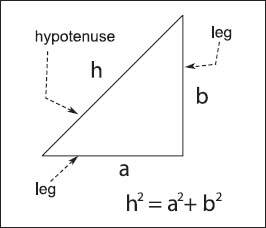NextPrevious

# What was Pythagoras’s importance to mathematics?The Pythagorean theorem is an easy way to determine the length of one side of a right triangle, given one knows the length of the other two sides.

Although the Chinese and Mesopotamians had discovered it over a thousand years before, most people credit the Greek mathematician and philosopher Pythagoras of Samos (c. 582-c. 507 B.C.E.) with being the first to prove the Pythagorean theorem. This is a famous geometry theorem relating the length of a right-angled triangle’s hypotenuse (h) to the lengths of the other two sides (a and b).

In other words, for any right triangle, the square of the length of the hypotenuse is equal to the sum of the squares of the lengths of the other two sides.

Close

This is a web preview of the "The Handy Math Answer Book" app. Many features only work on your mobile device. If you like what you see, we hope you will consider buying. Get the App